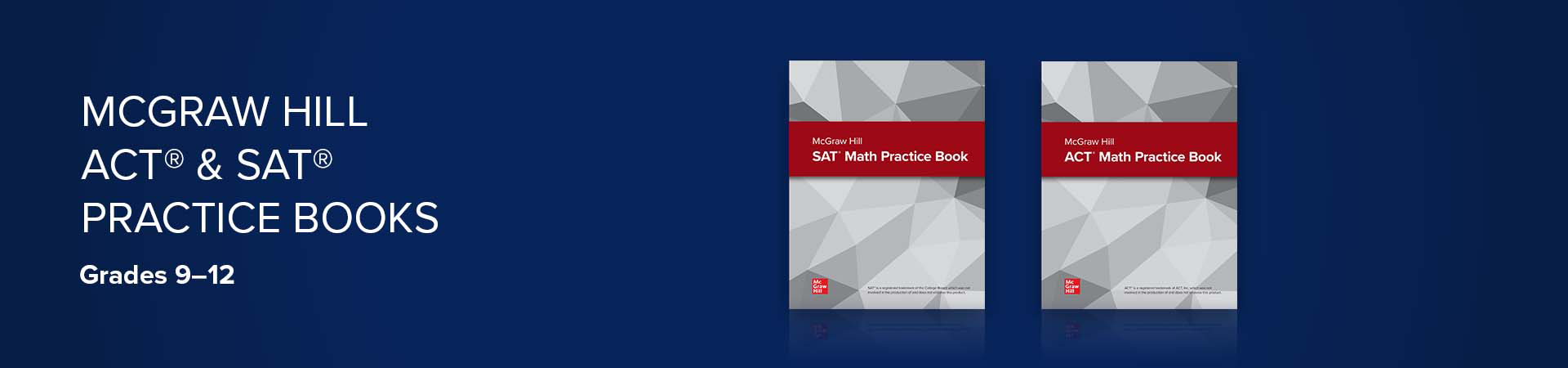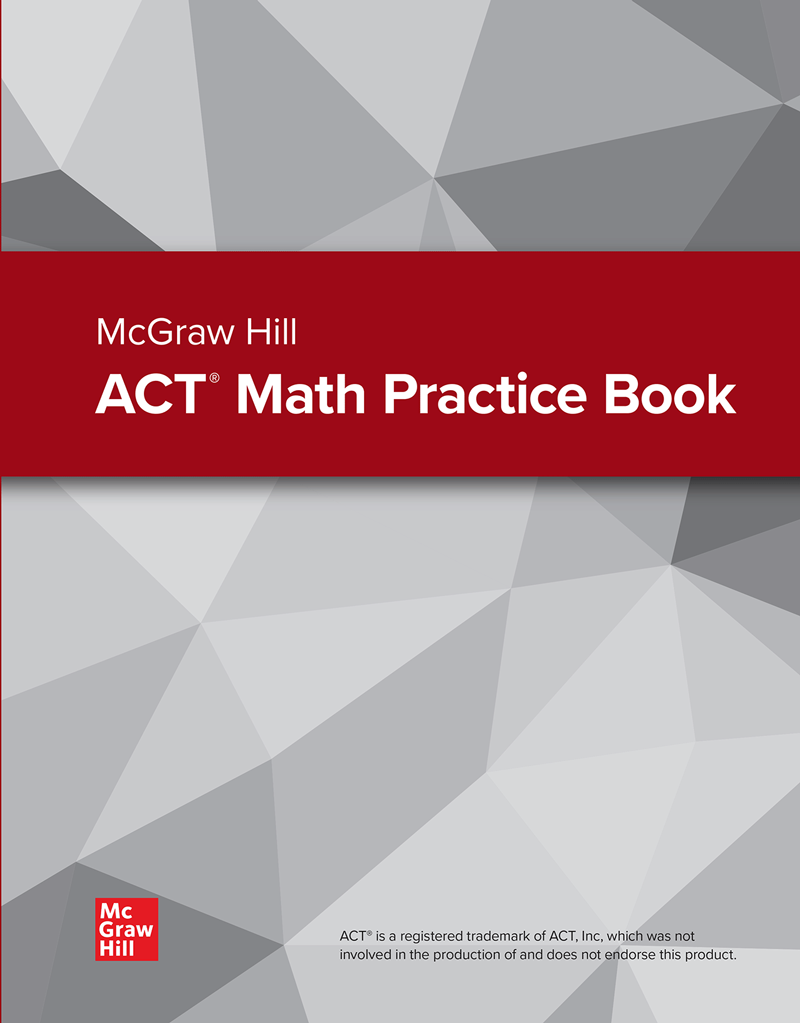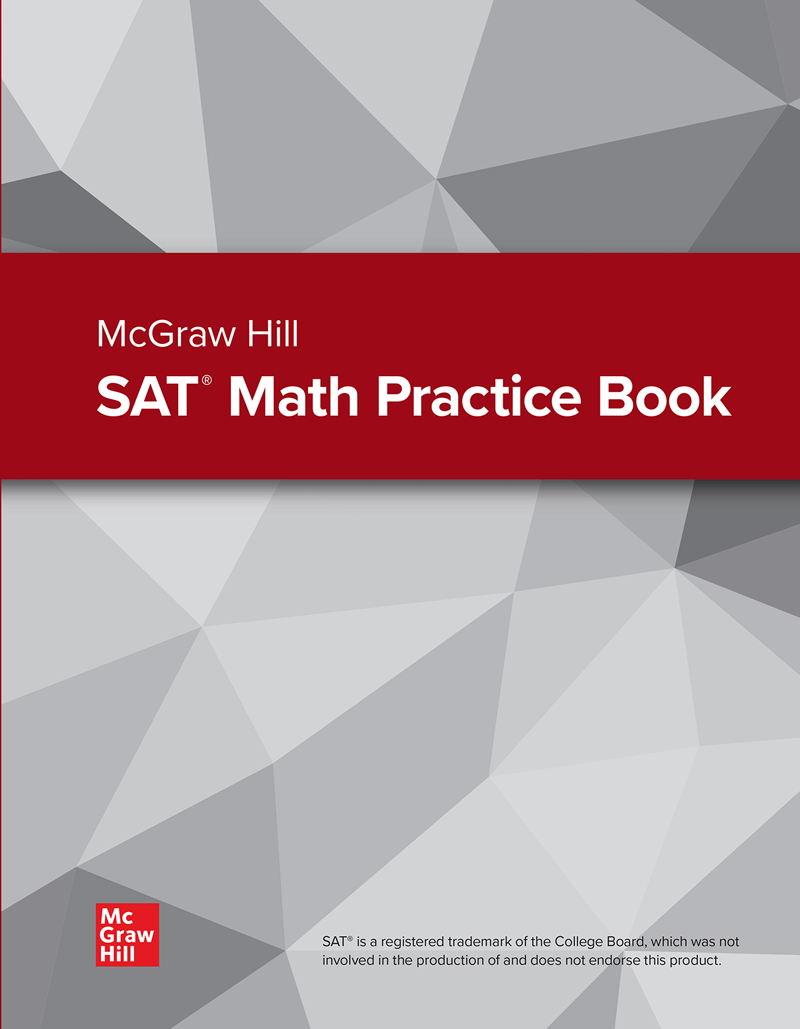## Prepare Your Students for the ACT® and SAT®

Introducing the McGraw Hill ACT® Math Practice Book and the McGraw Hill SAT® Math Practice Book. Designed to complement any high school math course, each resource provides students with structured practiced opportunities that focus specifically on the concepts and question types found on their respective test.

Each consumable book includes two practice tests along with practice concepts and answer keys.

McGraw Hill ACT® Math Practice Book

The ACT® Practice Book includes two pages of practice on the eight reporting categories:

• Preparing for Higher Math
• Number & Quantity
• Algebra
• Functions
• Geometry
• Statistics & Probability
• Integrating Essential Skills
• ModelingMcGraw Hill SAT® Math Practice Book

The SAT® Practice Book includes two pages of practice drawn from four math content categories:

• Problem Solving & Data Analysis